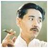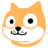首页   注册   登录
V2EX = way to explore
V2EX 是一个关于分享和探索的地方

V2EX  ›  前端开发

# 通过分析 AST 自动重构 three.js 的老旧代码

hujiulong · 311 天前 · 725 次点击

## 前言

three.js 曾经所有的文件都是使用全局变量`THREE`的方式来组织，比如欧拉角`Euler.js`

``````// three.js/src/math/Euler.js
THREE.Euler = function ( x, y, z, order ) {
this._x = x || 0;
this._y = y || 0;
this._z = z || 0;
this._order = order || THREE.Euler.DefaultOrder;
};
``````

## 为什么要用 AST 来做

1.正则要求很严格，每一个字符都要写规则来匹配它 如果代码风格不统一，例如想匹配`THREE.XX = xx`这种代码，你写的正则必须要同时兼容`THREE.XX=xx`这种等号两边没有空格的情况。实践中还要处理各种特殊情况，非常麻烦。

2.很难避开注释中的代码 注释中也可能会出现你要匹配的字符串，会导致很多错误。

JS 的 AST 已经形成了一套规范，具体可以看这个文档

## 找出输出语句

``````THREE.Euler = function() { /* ... */ };
``````

``````const Euler = function() { /* ... */ };
export { Euler };
``````

``````THREE.A = 1;
``````

``````{
"type": "AssignmentExpression",
"start": 1,
"end": 12,
"operator": "=",
"left": {
"type": "MemberExpression",
"start": 1,
"end": 8,
"object": {
"type": "Identifier",
"start": 1,
"end": 6,
"name": "THREE"
},
"property": {
"type": "Identifier",
"start": 7,
"end": 8,
"name": "A"
},
"computed": false
},
"right": {
"type": "Literal",
"start": 11,
"end": 12,
"value": 1,
"raw": "1"
}
}
``````

``````walk.simple( ast, {
AssignmentExpression: ( node ) => {
if (node.left.type === 'MemberExpression' &&
node.left.object.name === 'THREE') {
const { start, end, property } = node.left;
code.overwrite( start, end, `const \${property.name}` );  // 将 THREE.XX = xx 替换为 const XX = xx
exportVars.push(property.name);  // 将输出的变量保存，最后 export 它们
}
}
})
``````

## 处理依赖

``````const v = new THREE.Vector3();
``````

``````import { Vector3 } from 'three';
const v = new Vector3();
``````

``````walk.simple( ast, {
MemberExpression: node => {
const { object, property } = node;
if ( object.name === 'THREE' && property.type === 'Identifier' ) {
code.overwrite(object.start, object.end + 1, ''); // 将代码中的 THREE.XX 替换为 XX
dependences.push( property.name );  // 得到依赖
}
}
})
``````

## 后话

2 回复  |  直到 2019-03-19 10:43:03 +08:001 dandycheung   311 天前 via Android 赞。2 fy   311 天前 哇 大佬